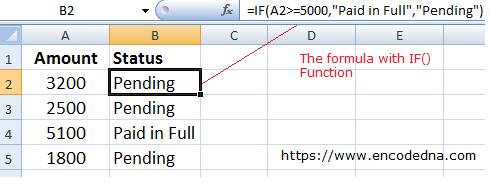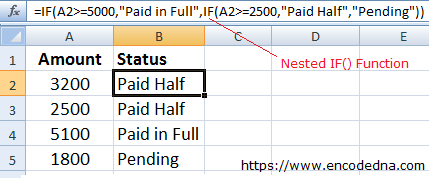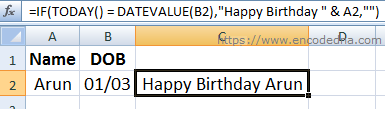﻿ How to use IF and Nested IF Functions in Excel

# How to use IF and Nested IF Functions in Excel

You can use the IF function in Excel for logical comparisons. This popular built-in function can also used in formulas with other functions. I am sharing few simple examples here in this post explaining how to use IF and Nested IF functions in Excel as formulas on text, numbers etc. and will show how to use these functions along with other function.

## The IF Syntax

IF(logical_test, [value_if_true], [value_if_false] )

The IF() function takes three parameters.

logical_test: The first parameter is the logical_test or in simple words, it means, If something is true. Its a condition, for example …

IF(A5>=5000, …

value_if_true: This is the second parameter, which says, do this if the logical_test is true.

value_if_false: The third parameter will execute if the logical_test returns false.

Let us now see some examples. The first example shows the basic usage of IF() function and second example shows how to apply nested IF() function in a Cell.

## The IF Function in Excel

I have two columns, Amount and Status. See the image below. In the first column, I would enter some numeric values as Amount. The second column Status will have a formula that would check the amount in the first column and accordingly show a message.

I’ll use the IF() function to check if the Amount entered meets the condition, that is, if the amount >= 5000, then show a message Paid in Full. Else, the message shows a status as Pending.I have five rows of data. In the second column I have applied a formula using the IF() function. The function checks the value in the first column of each row.

=IF(A2>=5000,"Paid in Full","Pending")

Simply write the above condition in the second column of the second row and later drag the second column down until the last row (with a value).

## Nested IF Function Example

Now, there will be situations, when you will need multiple conditions. I am extending the above example and add another condition.

If my users have paid 50% percent (or half) the amount, then it should notify me with another message, which says Paid Half.

=IF(A2>=5000,"Paid in Full",IF(A2>=2500,"Paid Half","Pending"))I have added a second IF() function as the False value for the first method. In the above example, the first two rows shows the status as Paid Half as it falls under the second IF() condition.

Related: The correct formula to Calculate Sales Percentage in Excel
Points to Remember

1) You do not have to start the second IF() function with an equal to (=) sign.
2) In Excel 2007 and above, you can add up to 64 IF() functions.

## Using IF() Function with Strings and Other Functions

You can concatenate string values inside the IF() function. Also, you can add other functions inside the IF() as conditions.

For example, I want to write a formula that will wish Happy Birthday to my user after checking the current Date, with a given Birth date. I have the name of the user and birth date in two different cells. Here’s what I’ll do.

=IF(TODAY() = DATEVALUE(B2),"Happy Birthday " & A2,"")I am using the TODAY() function to check the date in the cell B2. If the condition is true, that is, if today’s date is equal to the date in the second cell, wish the user, Happy Birthday. I have concatenated the string with value in the first cell.

I have not set any value as the false condition. You may add any condition you want.